Check the below Online Education NCERT MCQ Questions for Class 7 Maths Chapter 10 Practical Geometry with Answers Pdf free download. MCQ Questions for Class 7 Maths with Answers were prepared based on the latest exam pattern. We have provided Practical Geometry Class 7 Maths MCQs Questions with Answers to help students understand the concept very well. https://ncertmcq.com/mcq-questions-for-class-7-maths-with-answers/

Students can also refer to NCERT Solutions for Class 7 Maths Chapter 10 Practical Geometry for better exam preparation and score more marks.

## Online Education for Practical Geometry Class 7 MCQs Questions with Answers

Practical Geometry Class 7 MCQ Question 1.
In ΔRST, R = 5 cm, and ∠SRT = 45° and ∠RST = 45°. Which criterion can be used to construct ΔRST?
(a) A.S.A. criterion
(b) S.A.S. criterion
(c) S.S.S. criterion
(d) R.H.S. criterion

Hint: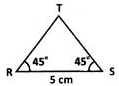Clearly, from the figure two angles and the included side are given. So, A.S.A. criterion can be used to construct ARST.

MCQ On Practical Geometry Class 7 Question 2.
Identify the criterion of construction of the equilateral triangle LMN given LM = 6 cm.
(a) S.A.S. criterion
(b) R.H.S. criterion
(c) A.S.A. criterion
(d) S.S.S. criterion

Hint:
Since ALMN is equilateral the measurement of one side is used for the other two sides of the triangle. Hence ALMN can be constructed by S.S.S. criterion.

Class 7 Maths Chapter 10 MCQ With Answers Question 3.
The idea of equal alternate angles is used to construct which of the following?
(a) A line parallel to a given line
(b) A triangle
(c) A square
(d) Two triangles

Answer: (a) A line parallel to a given line.

Class 7 Maths Chapter 10 MCQ Question 4.
A Given AB = 3 cm, AC = 5 cm,and ∠B = 30°, ΔABC cannot be uniquely constructed, with AC as base, why?
(a) Two sides and included angle are given.
(b) The other two angles are not given.
(c) The vertex B cannot be uniquely located.
(d) The vertex A coincides with the vertex C.

Answer: (c) The vertex B cannot be uniquely located.

MCQ On Pythagoras Theorem Class 7 Question 5.
A line panda point X not on it are given. Which of the following is used to draw a line parallel to p through X?
(a) Equal corresponding angles.
(b) Congruent triangles.
(c) Angle sum property of triangles.
(d) Pythagoras’ theorem.

Hint:
Corresponding angles of parallel lines are equal.

MCQ On Construction Of Triangles Class 7 Question 6.
Δ PQR is such that ∠P = ∠Q = ∠R = 60° which of the following is true?
(a) Δ PQR is equilateral.
(b) Δ PQR is acute angled.
(c) Both [a] and [b]
(d) Neither [a] nor [b]

Answer: (c) Both [a] and [b]
Hint:
In ΔPQR since all the angles are acute, it is acute angled. Also since all the angles are equal, it is equilateral.

Ncert Class 7 Maths Chapter 10 MCQ Question 7.
Which vertex of ΔABC is right angled if $$\overline { AB }$$ = 8 cm, $$\overline { AC }$$ = 6 cm,and $$\overline { BC }$$ = 10 cm,?
(a) ∠C
(b) ∠A
(c) ∠B
(d) A or C

Hint:
From the given measurements, $$\overline { BC }$$ is the hypotenuse. The angle opposite to $$\overline { BC }$$ is ∠A which is a right angle.

MCQ On Geometrical Construction Class 7 Question 8.
An isosceles triangle is constructed as shown in the figure.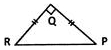Which of the given statements is incorrect?
(a) $$\overline { PR }$$ is the hypotenuse of ΔPQR.
(b) ΔPQR is an equilateral triangle.
(c) ΔPQR is a right angled triangle.
(d) If right angled ΔPQR has its equal angles measuring 45° each.

Answer: (b) ΔPQR is an equilateral triangle.

Class 7 Practical Geometry MCQ Question 9.
ΔPQR is constructed with all its angles measuring 60° each. Which of the following is correct?
(a) ΔPQR is an equilateral triangle.
(b) ΔPQR is isosceles triangle.
(c) ΔPQR is a scalene triangle.
(d) ΔPQR is a right angled triangle.

Answer: (a) ΔPQR is an equilateral triangle.

Practical Geometry MCQ Class 7 Question 10.
How many perpendicular lines can be drawn to a line from a point not on it?
(a) 1
(b) 2
(c) 0
(d) Infinite

Hint: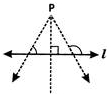As can be seen from the given figure, one and only one perpendicular line can be drawn to a given line from a point not on it.

Class 7th Maths Chapter 10 MCQ Question 11.
Identify the false statement.
(a) A triangle with three equal sides is called an equilateral triangle.
(b) A triangle with a right angle is called a right angled triangle.
(c) A triangle with two equal sides is called a scalene triangle.
(d) A right angled triangle has two acute angles and a right angle.

Answer: (c) A triangle with two equal sides is called a scalene triangle.

Class 7 Maths Ch 10 MCQ Question 12.
ΔPQR is constructed such that PQ = 5 cm, PR = 5 cm and ∠RPQ = 50° Identify the type of triangle constructed.
(a) An isosceles triangle
(b) An acute angled triangle
(c) An obtuse angled triangle
(d) Both [a] and [b]

Answer: (d) Both [a] and [b]

Class 7 Maths Chapter 10 Extra Questions Question 13.
Which of the following is NOT constructed using a ruler and a set square?
(a) A perpendicular to a line from a point not on it.
(b) A perpendicular bisector of a line segment.
(c) A perpendicular to a line at a point on the line.
(d) A line parallel to a given line through a given point.

Answer: (b) A perpendicular bisector of a line segment.

Question 14.
Study the steps of construction given.
Step 1: Draw a ray OA.
Step 2: With O as centre and any convenient radius draw an arc MN to cut OA at M.
Step 3: With M as centre and the same radius draw an arc to cut MN at P.
Step 4: With P as centre and the same radius, draw an arc to cut MN at Q.
Step 5: Draw OQ and produce it to D. An angle AOD is constructed.
What is the measure of ∠AOD?
(a) 60°
(b) 30°
(c) 120°
(d) 45°

Hint:
The given steps of construction are to construct an angle of 120°.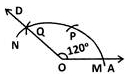Question 15.
In ΔXYZ, x, y and z denote the three sides. Which of the following is incorrect’?
(a) x – y > z
(b) x + z > y
(c) x – y < z
(d) x + y > z

Answer: (a) x – y > z
Hint:
The difference of two sides of a triangle is less than its third side.

Question 16.
In which of the following cases can a triangle be constructed?
(a) Measures of three sides are given.
(b) Measures of two sides and an included angle are given.
(c) Measures of two angles and the side between them are given.
(d) All the above.

Question 17.
Based on the sides of a triangle, which of the following is a classification of triangles?
(a) A right angled triangle
(b) An acute angled triangle
(c) An obtuse angled triangle
(d) An isosceles triangle

Question 18.
Which of the following is used to draw a line parallel to a given line?
(a) A protractor
(b) A set square
(c) A ruler
(d) A ruler and compasses

Answer: (d) A ruler and compasses
Hint:
A line parallel to a given line can be drawn using a ruler and a compass.

Question 19.
Direction: David folds a sheet of paper. The dotted lines as shown in the figure are the creases formed, which are named as l, m and n.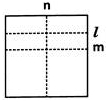What can you say about lines I and n?
(a) l // n
(b) l ⊥ n
(c) I is the same line as n
(d) Neither [a] nor [b]

Hint:
A 90° angle is formed at the intersection of l and n. So l ⊥ n.

Question 20.
A Choose the correct option in which a triangle CANNOT be constructed with the given lengths of sides.
(a) 3 cm, 4 cm, 5 cm
(b) 7 cm, 6 cm, 5 cm
(c) 10 cm, 7 cm, 2 cm
(d) 12 cm, 8 cm, 6 cm

Answer: (c) 10 cm, 7 cm, 2 cm
Hint:
The difference of any two sides of a triangle must be less than the third side. This property of triangles is not satisfied by the given measurements as 10 – 7 = 3 > 2 and 10 – 2 = 8 > 7, though 7 – 2 = 5 < 10 is true.

Question 21.
Identify the true statement.
(a) A triangle with 3 equal sides is isosceles.
(b) A triangle with a 110° angle is right angled.
(c) A triangle with 3 acute angles is acute angled.
(d) A triangle with 2 equal sides is equilateral.

Answer: (c) A triangle with 3 acute angles is acute angled.

Question 22.
Which of the following statements is incorrect?
(a) The sum of angles in a triangle is 2 right angles.
(b) The exterior angle of a triangle is equal to the interior angle of the triangle.
(c) The hypotenuse is the longest side of a right angled triangle.
(d) All the above.

Answer: (b) The exterior angle of a triangle is equal to the interior angle of the triangle.

Question 23.
A triangular sign board is isosceles. If the unequal side is 7 cm and one of the equal sides is 6 cm, what is the measure of the third side?
(a) 5 cm
(b) 6 cm
(c) 7 cm
(d) Either [a] or [c]

Question 24.
In the given figure, find the measure of ∠ROT, if PQ = QR and ∠QPR = 60°.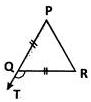(a) 60°
(b) 140°
(c) 120°
(d) 100°

Hint:
ΔPQR is isosceles since PQ = QR.
∴ ∠QPR = ∠QRP = 60° ∠RQT is the exterior angle of ΔPQR which is equal to the sum of interior opposite angles ∠P and ∠R.
Hence, ∠RQT = 60° + 60° = 120°.

Question 25.
Which among the following is used to construct a triangle?
(a) The lengths of the three sides.
(b) The perimeter of the triangle.
(c) The measures of three angles.
(d) The names of three vertices.